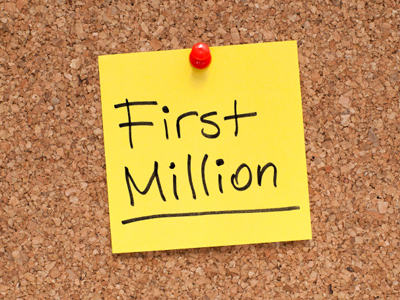10,000 × 100 = 1,000,000.

# Multiplication and Division (Difficult)

Symbols are used for multiplication and division in mathematical equations. The x denotes multiplication and the ÷ denotes division. This 11-plus Maths quiz is the first in our series of difficult quizzes on multiplication and division. Remember to look at all four answers before making your choice. Here's a reminder of two useful tips:

1. To multiply a number by another number which is a multiple of 10 and whose only non zero digit is the leading digit 1: move the digits to the left by the same number of zeros that are in the multiple of 10. Note: whole numbers that do not have decimal parts are written without a decimal point e.g. 400.0 = 400: in such cases the d.p. is not normally needed.
2. To divide a number by another number which is a multiple of 10 and whose only non zero digit is the leading digit 1: move the digits to the right by the same number of zeros that are in the multiple of 10.
1.
What is the correct answer to the given calculation?
18.56 × 100 = ?
0.1856
185.6
1,856
18,560
TIP 1. Move the d.p. 2 places to the right to give 1,856
2.
What is the correct answer to the given calculation?
2,500 ÷ 1,000 = ?
0.25
2.5
25
250
TIP 2. 2,500 = 2,500.0 Move the d.p. 3 places to the left to give 2.5
3.
What is the correct answer to the given calculation?
199.09 × 1,000 = ?
19,909
199,090
0.19909
1,990,900
TIP 1. Move the d.p. 3 places to the right to give 199,090
4.
What is the correct answer to the given calculation?
0.124 ÷ 100 = ?
12.4
1.24
0.00124
0.0124
TIP 2. Move the d.p. 2 places to the left to give 0.00124
5.
What is the correct answer to the given calculation?
10,000 × 100 = ?
10,000,000
10,000
100,000
1,000,000
TIP 1. 10,000 = 10,000.0 Move the d.p. 2 places to the right to give 1,000,000
6.
What is the correct answer to the given calculation?
250,000 ÷ 10,000 = ?
25,000
2,500
250
25
TIP 2. 250,000 = 250,000.0 Move the d.p. 4 places to the left to give 25
7.
What is the correct answer to the given calculation?
123.608 × 100 = ?
123,608
1,236.08
12,360.8
1.23608
TIP 1. Move the d.p. 2 places to the right to give 12,360.8
8.
What is the correct answer to the given calculation?
1.11 ÷ 100 = ?
111
0.0111
0.00111
11.1
TIP 2. Move the d.p. 2 places to the left to give 0.0111
9.
What is the correct answer to the given calculation?
7.777 × 100 = ?
777.7
7,777
77.77
0.07777
TIP 1. Move the d.p. 2 places to the right to give 777.7
10.
What is the correct answer to the given calculation?
34,567 ÷ 10,000 = ?
3.4567
3,456.7
0.34567
0.034567
TIP 2. 34,567 = 34,567.0 Move the d.p. 4 places to the left to give 3.4567
Author:  Frank Evans

We use cookies to make your experience of our website better.

To comply with the new e-Privacy directive, we need to ask for your consent -